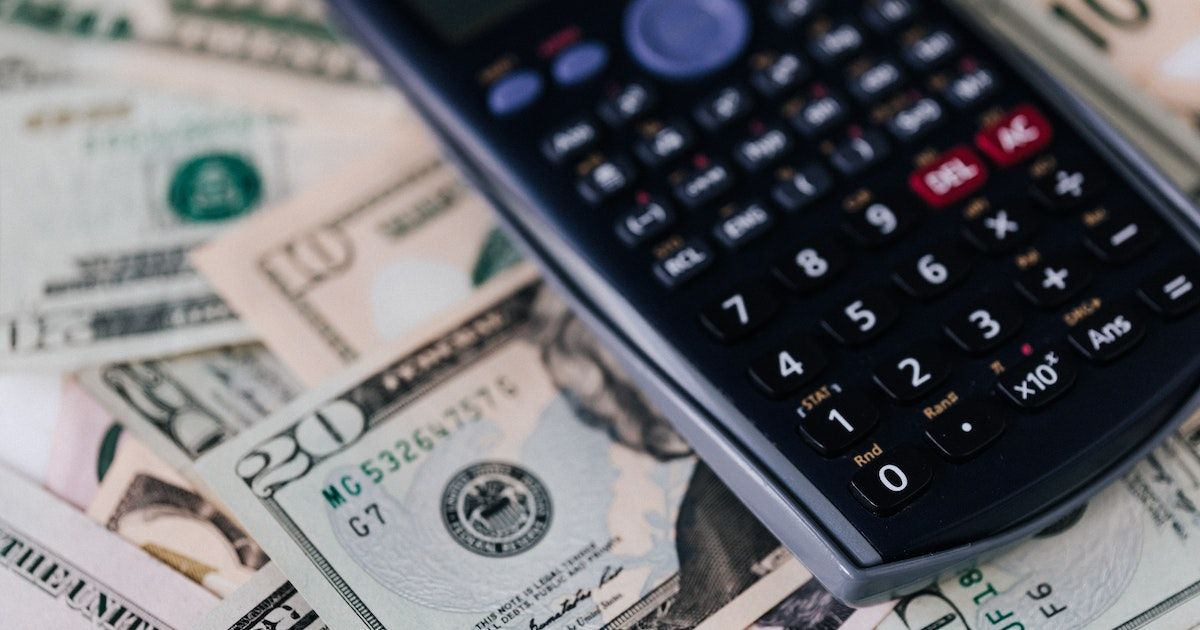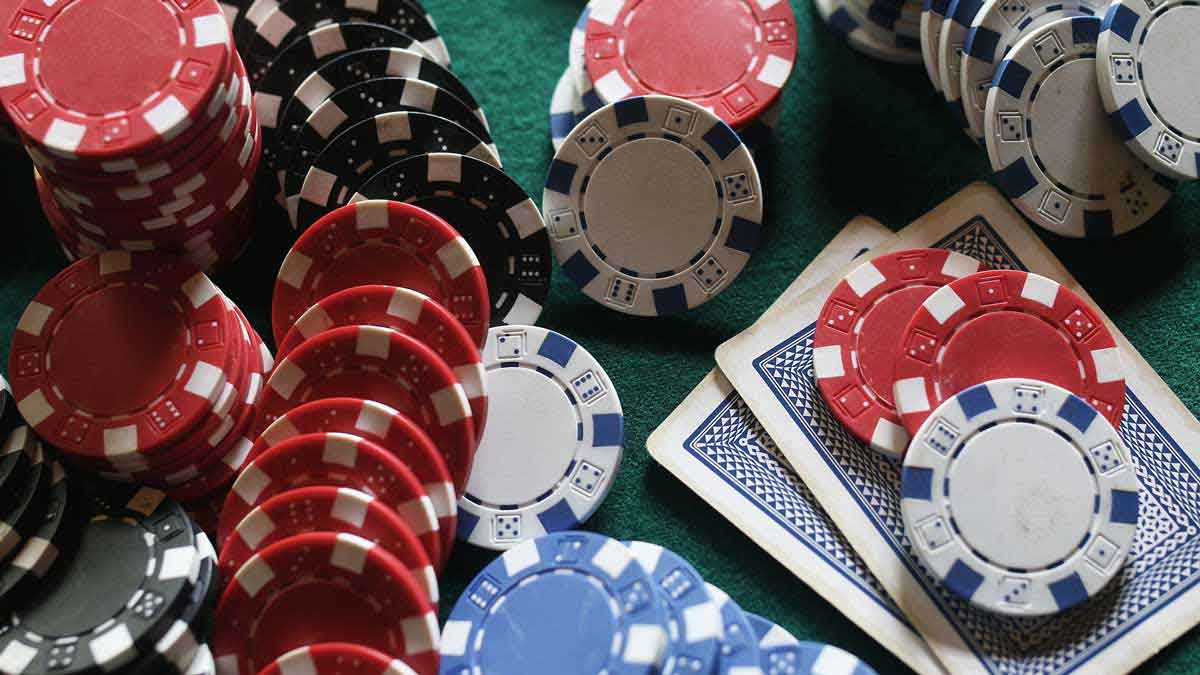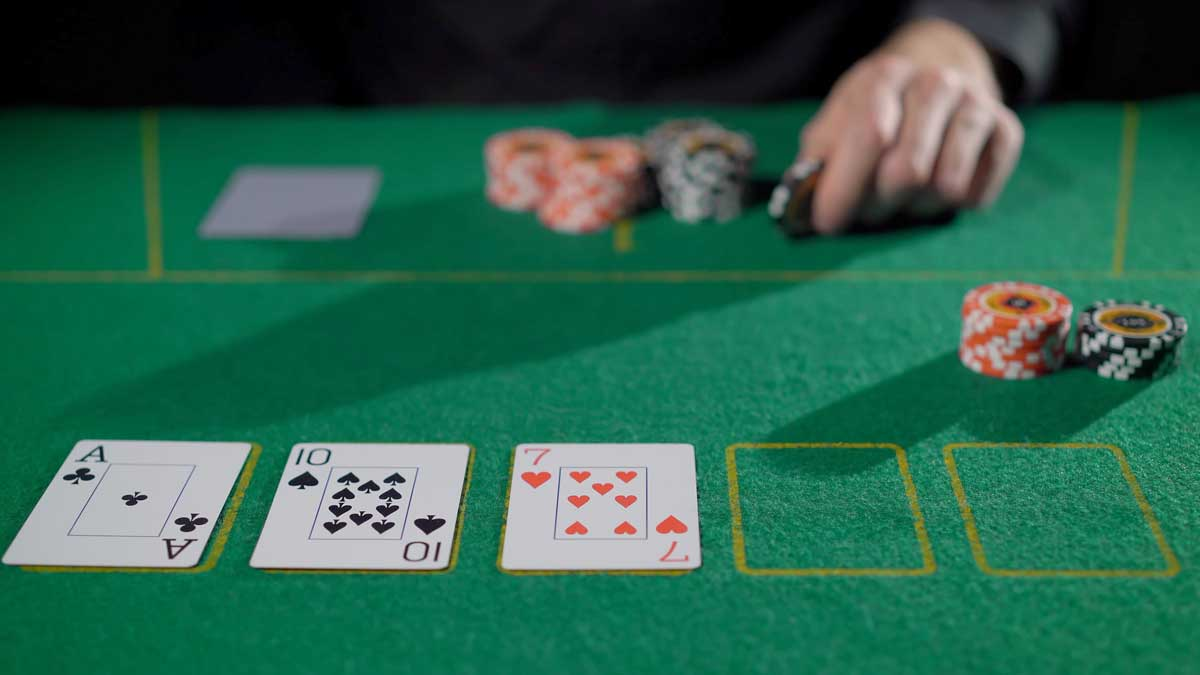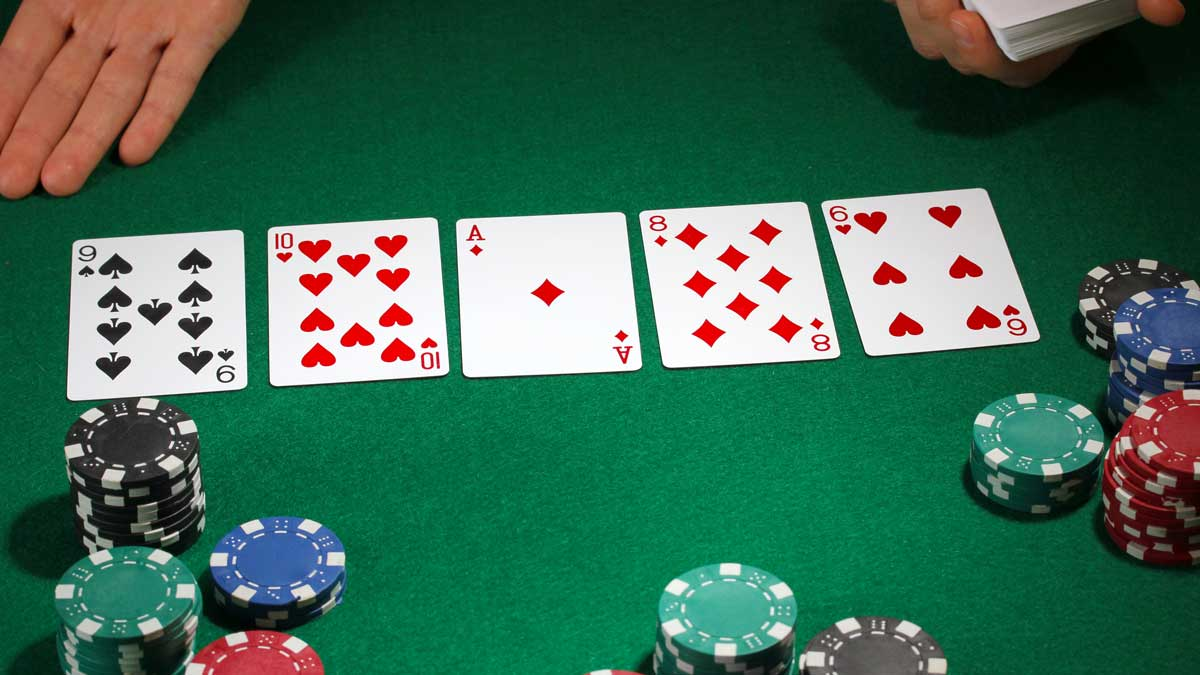# How To Calculate Pot Odds When Playing Poker

Dominic Field

May 23, 2023Pot odds are an extremely important concept in poker. In fact, it’s the basis for every single call ever made. Without pot odds, we’d never be able to understand which calls are profitable and which are not. In this article, we’ll explain how to calculate your pot odds and discuss other issues such as equity and implied odds.

## Poker Probability

Before we jump straight to the main topic of this article, let's shine some light on poker probability. In a standard deck of 52 cards, two cards are dealt to each player before the flop, leaving 50 unknown cards that could appear on the flop. This means that the odds of a specific card appearing on the flop are 1/50 or 2%.

As the game progresses, more cards are revealed, and the odds of a specific card appearing change. For example, on the turn, there are three more known cards (the flop), so the odds of a specific card appearing are 1/47 or 2.12%. Similarly, on the river, there are four known cards, so the odds of a specific card appearing are 1/46 or 2.17%. Assigning each card a 2% chance of appearing on any street is a useful approximation for quick calculations.

## What Are Pot Odds?

“Pot odds” refers to the amount you’ll receive if you win the pot, in relation to what you need to pay in order to call a bet. Basically, betting in poker is no different from placing a wager with a bookmaker or at a roulette table. But your payout is obviously determined by whatever is in the pot at the time.

But how do we calculate poker odds?

## Pot Odds Example

Let’s say there’s \$20 in the pot on the river. Your opponent is first to act and they bet out half the pot. You are now facing a \$10 bet and there is nobody left to act behind you.

If you make the call and win, you have invested \$10 in order to win a total of \$40. That’s a \$30 profit, plus your own \$10 call. In other words, you have to bet \$10 to win \$30, which is simplified as \$1 to win \$3. That’s a 3-to-1 odds.

## How to Use Pot OddsOkay, so now we understand how to calculate pot odds. But what do we do with this information? First of all, we need to link the odds to our actual hand. The most common example is when we are on some kind of draw.

For instance, let’s say after the turn, we have four diamonds and are drawing to the nut flush. We know that there are nine other diamonds in the deck that will complete our hand. With 6 cards revealed to us, there are 46 left and 9 of them will improve our hand. That means almost 20% of the deck helps us.

If 1 in every 5 cards gives us the win, 4 in every 5 do not. That’s odds of 4 to 1. So if our pot odds are offering 4 to 1, we can justifiably make the call and draw to our flush. If the pot odds are even greater, we’re getting tremendous value. If the pot odds are anything less, we know this is not a profitable long-term play.

## Calculating Outs And Equity

In order to make these calculations, you need to understand which cards are needed to improve your hand. It’s not always easy to know what your outs are, since poker involves a lot of hidden information. Sometimes you think a card is an out, but it actually helps our opponent too.

As a general rule, though, the following poker odds chart will help cover the most common scenarios:

Flush Draw 9 Outs
Open-Ended Straight Draw 8 Outs
Inside Straight Draw 4 Outs
Two Overcards 6 Outs
Flush Draw Plus One Overcard 12 Outs
Flush Draw Plus Two Overcards 15 Outs

If you prefer to think in terms of equity, the rule of thumb is this; each additional out provides around a 4% chance of hitting on the turn and river. So if you have ten outs, your chances of winning are roughly 40%.

## Calculating Pot Odds as a Percentage

Here’s how to calculate pot odds as a percentage.

1. Work out the total pot size after you make the call.

2. Divide the call amount by the pot amount.

3. Multiply the figure by 100.

So if you are facing a \$5 bet into a pot of \$2:

1. Total pot size = \$25

2. 5 / 25 = 0.20

3. 0.20 * 100 = 20%

## Antiouts And Blockers

Antiouts and Blockers are two important concepts to consider when calculating your outs in poker. Antiouts are cards that can improve your hand but also improve your opponent's hand. For example, if you have a flush draw, and there is a straight draw on the board, any card that completes the straight would be an antiout for you.

On the other hand, blockers are cards that reduce the number of outs available to your opponent. For instance, if you hold the Ace of Hearts and the King of Hearts, you are blocking your opponent from having the nut flush draw. Understanding the significance of antiouts and blockers can help you make better decisions when playing poker, as it allows you to assess the strength of your hand and your opponent's hand more accurately.

## Calculating Texas Hold'em Poker OddsHow to calculate poker odds for Texas Hold'em? It's simple. First, you need to know the number of outs you have, which are the cards that can improve your hand.

For example, if you have a flush draw, you have nine outs to complete your flush. To calculate your odds of hitting your flush, you need to multiply the number of outs by two and add two. In this case, your odds of hitting your flush on the next card are approximately 19%. Another common scenario is when you have an open-ended straight draw, which means you have eight outs to complete your straight. In this case, your odds of hitting your straight on the next card are approximately 17%.

## Examples of Profitable and Unprofitable Pot Odds

### Profitable Pot Odds

Let's see, using real examples, how can we evaluate our pot odds? Let's say you're playing Texas Hold'em, and you have a hand of 8-9. The flop comes out as 6-7-J, giving you an open-ended straight draw and a flush draw. Your opponent bets \$20 into a \$30 pot, and you need to decide whether to call or fold.

To calculate your pot odds, you divide the amount you need to call (\$20) by the total pot size (\$50, which is the \$30 pot plus the \$20 bet). This gives you pot odds of 40%.

Next, you need to calculate your odds of making your hand. You have 15 outs (four 5s, four 10s, and four spades), which gives you a 54% chance of making your hand by the river.

Since the pot odds (40%) are lower than your odds of making a hand (54%), this is a profitable call. In other words, you expect to win more money in the long run by calling in this situation than by folding.

## Unprofitable Pot OddsA good example of unprofitable pot odds is as follows. You have a hand of Q-J. The flop comes out as 9-T-A, giving you an open-ended straight draw. Your opponent bets \$20 into a \$30 pot, you need to decide whether to call or fold.

To calculate your pot odds, you divide the amount you need to call (\$20) by the total pot size (\$50, which is the \$30 pot plus the \$20 bet). This gives you pot odds of 40%.

Next, you need to calculate your odds of making your hand. You have eight outs (four 8s and four Kings), which gives you a 16% chance of making your hand by the turn.

Since your pot odds (40%) are higher than your odds of making your hand (16%), this is an unprofitable call. You can expect to lose money by calling in this situation. Therefore, it would be better to fold your hand and wait for a better opportunity.

## Implied Odds

When we talk about pot odds, we are looking at a single decision in isolation. And of course, real games of poker are never that simple. It is often the case that you can be sure an opponent will give you further action later in the hand. The pot may not be laying the correct odds at this moment in time, but when you know you’re going to get a call later on, at a much bigger price, you can sometimes justify taking the wrong price.

For instance, let’s take a game of \$2/\$4 Limit Hold’em. You are facing a bet of \$4 into a \$16 pot. That’s pot odds of 5 to 1 (staking \$4 to win a total of \$20, or \$1 to win \$5). However, you may know your opponent is invested enough in the hand that they’ll definitely call another bet in the end. If that’s the case, you’re going to win another \$4, so your implied odds are 6 to 1. And if a raise is involved, it would rise to 7 to 1.

## Final Thoughts

Pot odds are one of the most important concepts to master. Without them, we would never understand what makes a profitable long-term call. Make sure you take the time to understand the idea and spend time practising. Your all-round game will thank you.

## Poker Odds FAQs

#### Q: Can you calculate poker odds?

Yes, you can calculate poker odds. To calculate poker odds, you need to know the number of outs you have, which are the cards that can improve your hand. Once you know the number of outs, you can calculate your odds of hitting your hand by using a simple formula. The formula is (number of outs/number of unseen cards) x 100.

For example, if you have a flush draw with nine outs and there are 47 unseen cards, your odds of hitting your flush on the next card are approximately 19%. It's important to note that this is just a rough estimate, and your actual odds may vary depending on the specific situation.

Additionally, calculating poker odds is just one aspect of playing poker, and there are many other factors to consider when making decisions at the poker table.

#### Q: What is the easiest way to calculate odds?

The easiest way to calculate odds in poker is to use the rule of 2 and 4. This rule is a quick and easy way to estimate your odds of hitting your hand on the next card or on the turn and river combined. To use the rule of 2 and 4, you simply multiply your number of outs by 2 or 4, depending on whether you are calculating your odds on the next card or on the turn and river combined.

#### Q: Do poker odds change with more players?

Yes, poker odds can change with more players. The more players there are in a hand, the more likely it is that someone has a strong hand, which can affect your odds of winning.

Additionally, the more players there are, the more likely it is that someone will have the same outs as you, which can reduce your odds of hitting your hand. For instance, if you have a flush draw with nine outs and there are two other players in the hand, it's more likely that one of them has a flush draw as well, which reduces your odds of hitting your flush. It's important to consider the number of players in a hand when calculating your odds.

#### Q: What are the best odds in poker?

The best odds in poker are when you have a made hand, which means you already have a strong hand without needing to improve it. If you have a pair of Aces, your odds of winning the hand are very high, especially if there are no other Aces on the board. Another example of a strong made hand is a straight or a flush.

These hands have very good odds of winning, especially if they are the highest possible straight or flush. However, it's important to note that the best odds in poker can vary depending on the specific situation and the number of players in the hand. Having the best odds doesn't guarantee that you will win the hand, as there are many other factors to consider when you play poker.

#### Q: What is the 2/4 rule in poker?

The rule states that if you multiply the number of outs you have by 2, it will give you an approximate percentage of your odds of hitting your hand on the next card. If you multiply the number of outs by 4, it will give you an approximate percentage of your odds of hitting your hand by the river (the last card dealt in Texas Hold'em).

For example, if you have a flush draw with 9 outs, the 2/4 rule would estimate your odds of hitting your flush on the turn (the next card) at approximately 18% (9 x 2 = 18) and your odds of hitting your flush by the river at approximately 36% (9 x 4 = 36). It's important to note that the 2/4 rule is just an estimate, and your actual odds may vary depending on the specific situation.